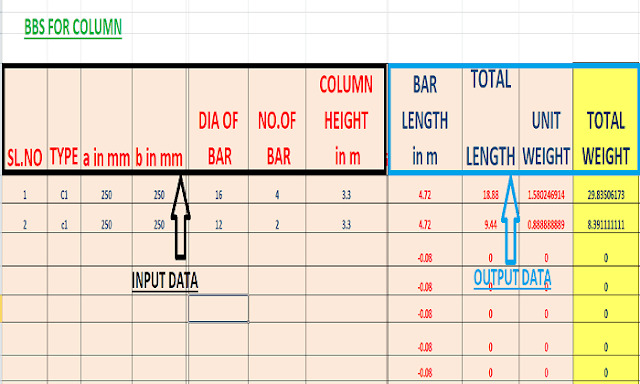## What is Bar Bending Schedule? | Advantages Bar Bending Schedule

Are you struggling at  Bar Bending Schedule? Learn the advantages of bar-bending-schedule.
Here I shared, How to Calculate Steel quantity in Bar Bending Schedule.

### What is Bar Bending Schedule?

Bar Bending Schedule is basically helping us to easily visualize the required steelwork for a particular reinforced concrete work or any work item in which steel is used.

As a civil engineer, Many times you need to calculate all the kinds of stuff that are related to steelwork i.e diameter of the bar, cutting length of the bar, hook length, stirrup details, the total length of the bar, the total weight of the bar, etc.

That's a very lengthy and complicated process.

THIS IS WHY WE LOVE BAR-BENDING-SCHEDULE (BBS).

Advantages of bar bending schedule are simply unavoidable.

BBS is a tabular form that will give you a clear image of steel-related work(including reinforced concrete).

Before starting the Bar-bending-schedule tutorial, let us learn some basic knowledge that will help you throughout this tutorial.

#### 1.Minimum clear cover

• SLAB- 15 mm
• BEAM- 25 mm
• COLUMN- 40 mm
• FOOTING- 50 mm
• PILE FOUNDATION- 75 mm

#### 2.Standard length-

• Hook lenght= 6D OR 9D.
• Stirrup leg= 12D.
• Floor to floor height=3meter (approx.)
*(Where D is the diameter of the bar)

### 3.Bar bending schedule formula-

• Unit weight of steel=(d^2/162)kg/m(where d is in mm)
• Total weight of bar=[Unit weight(kg/meter)* total length of bar(meter)]kg
• No.of bar in an item=[(length of item/centre to centre  distance of bar)+1]

### How to calculate steel quantity

Let's do a quick example of BBS to know how this works-

So, as per the above image, we have a top view of a column.

Lets, find out the TOTAL STEEL QUANTITY OF STIRRUP WORK IN THIS COLUMN.

GIVEN DATA-

• COLUMN DIMENSION(230 * 375)mm
• VALUE OF A=a
• VALUE OF C=c
• (2A+2C)= PERIMETER OF STIRRUP WITHOUT STIRRUP LEG
• CONSIDERING LENGTH OF STIRRUP LEG=12*d(d=dia of stirrup bar)
• CONSIDERING,2 LEGGED STIRRUP IS USED.
• LETS CONSIDER DIA OF STIRRUP BAR= 8mm
• SPACING OF STIRRUP BAR=180mm
• column length = 4meter
ANS-

First, we have to calculate the total length of the stirrup.

the total length of the stirrup= perimeter of stirrup + length of the stirrup leg

HERE, length of stirrup=(2A+2C)=(2*140 + 2*285) mm=850mm

length of stirrup leg= (2*12*d)=192mm (multiplying by 2 because we are using 2 legged stirrups)

HENCE, Total length= 850+192=1042 mm

TOTAL NO. OF STIRRUP=column length/spacing=(4000/180)+1=24 nos

The total length of stirrup bar = no of the stirrup * length of each bar=24*1042=25 m

Unit Weight of stirrup bar = (d^2/162)=(8^2/162)=0.39 kg/m

Total weight = unit weight * total length = 25 * 0.39 = 9.75 kg

Hence,

The total quantity of steel = 9.75 kg

From this example, you get a basic idea about how to make BBS work.
In a similar manner, you can calculate the quantity of steel for any item work.

### BAR BENDING SCHEDULE FORMATStandard BBS Table for the column in excel

From the above image, you can see that, We have some input value and some output value.

I suggest you do the BBS work in excel.

You have to calculate all the stuff only one time in excel.
After that,you can get all the required value from just input data.

If you want the complete excel file of bar bending schedule then comment below.

Advantages of bar-bending schedule are simply enhanced by the excel format, as this simplifies our problem and calculation.

### ## ADVANTAGES OF BAR-BENDING-SCHEDULE

1. By using ng BBS method, you can have an actual idea of how much reinforcement is needed for a particular work or project.
2. BBS is very useful in estimating and costing.It gives you a proper estimation of cost regarding reinforcement.
3. This helps to minimize the wastage of reinforcement on the site.
4. As you get the predetermined amount of reinforcement, cutting of bar can be done in factory or any other place from where it can be transported to the site.
5. BBS makes the construction work less time consuming.

SUMMARY

From this article, you get a brief idea about the bar bending schedule and also about the advantages of bar bending schedule.
In simple words, Bar bending schedule is something that will help to simplify your calculation of steel or reinforcement. This tabular form of BBS is very known and widely used in civil engineering.

It has various of advantages that i discussed above. Bar bending schedule is very much inter connected with estimating and costing as BBS gives you an approximate amount that will be required for reinforcement only.

IF THIS POST FELT VALUABLE TO YOU FEEL FREE TO SHARE.

What is Bar Bending Schedule? | Advantages Bar Bending ScheduleReviewed by Sk rafiqul islam on August 17, 2018 Rating: 5